## Tiny Function Generator

18th February 2018

This article describes a simple function generator based on an ATtiny85. It can generate triangle, sawtooth, square, and rectangular waves, a pulse train, and noise. The frequency can be adjusted using a rotary encoder between 1Hz and 5kHz in steps of 1Hz, and the selected waveform and frequency is displayed on an OLED display: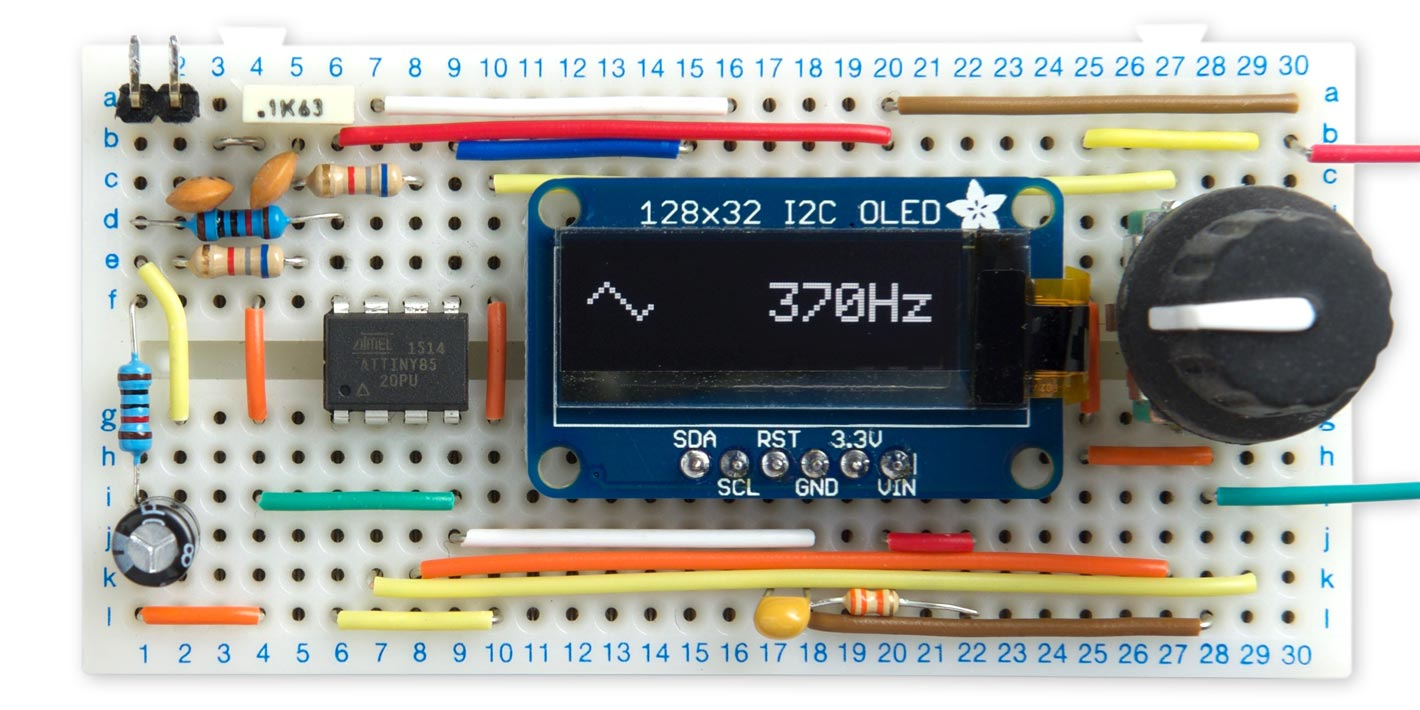The Tiny Function Generator based on an ATtiny85; it's generating a 370Hz triangle wave.

This project really puts the ATtiny85 through its paces; it's generating 8-bit samples at a 16kHz sampling rate, decoding the rotary encoder, switching between waveforms, and updating the OLED display via I2C.

The project is based on my earlier article Waveform Generation using an ATtiny85, and was inspired by a suggestion of Roberto Pangallo.

For an extension to this project that adds a sine wave see Tiny Function Generator Sine Wave.

I have designed a PCB for this circuit: see Tiny Function Generator PCB.

### Introduction

The Tiny Function Generator uses Direct Digital Synthesis or DDS to generate the signals. Usually DDS uses a table of a pre-computed waveform, such as a sine wave. To generate a particular frequency you step through the table selecting every nth sample. The smaller the number used to step, the longer it takes to get around one cycle of values, and so the lower the frequency.

The ATtiny85 is ideal for DDS as it has a special 64MHz clock option which you can use to drive Timer/Counter1 for fast digital-to-analogue conversion. Here's the routine to set up the timer/counters for DDS:

```void SetupDDS () {
// Enable 64 MHz PLL and use as source for Timer1
PLLCSR = 1<<PCKE | 1<<PLLE;

// Set up Timer/Counter1 for PWM output
TIMSK = 0;                               // Timer interrupts OFF
TCCR1 = 1<<PWM1A | 2<<COM1A0 | 1<<CS10;  // PWM A, clear on match, 1:1 prescale
pinMode(1, OUTPUT);                      // Enable PWM output pin

// Set up Timer/Counter0 for 20kHz interrupt to output samples.
TCCR0A = 3<<WGM00;                       // Fast PWM
TCCR0B = 1<<WGM02 | 2<<CS00;             // 1/8 prescale
TIMSK = 1<<OCIE0A;                       // Enable compare match, disable overflow
OCR0A = 60;                              // Divide by 61
}```

The first section turns on the 64MHz Phase-Locked Loop (PLL), and specifies this as the clock source for Timer/Counter1.

Timer/Counter1 is then set up in PWM mode, to make it act as an analogue-to-digital converter, using the value in OCR1A to vary the duty cycle and hence the analogue output. The frequency of the square wave is specified by OCR1C; we leave it at its default value, 255, which divides the clock by 256, giving a 250kHz square wave.

Timer/Counter0 is used to generate an interrupt to output the samples. The rate of this interrupt is the 8MHz system clock divided by a prescaler of 8, and a divisor of 61, giving about 16.4kHz. The interrupt calls an Interrupt Service Routine ISR(TIMER0_COMPA_vect) which calculates and outputs the samples.

Fortuitously, the divisor of 61 enables us to get a frequency step very close to 1Hz. For example, here's the calculation showing what frequency you get for a Jump value of 4. The Interrupt Service Routine is called once every 8000000/(8*61) Hz, and each time Jump is added into the 16-bit phase accumulator, Acc. The top bit of Acc will therefore change with a frequency of:

8000000/(8*61)/(65536/4) or 1.0006 Hz.

In summary: changing the value of Jump in steps of 4 will give us 1 Hz steps in frequency to better than 0.1% accuracy.

### Generating the waveforms

To avoid the need to calculate and store wavetables the Tiny Function Generator calculates the waveforms on the fly as described in the following sections. The code for each waveform avoids conditional statements to ensure that the execution time is consistent on each cycle.

#### Square

The square wave has a duty cycle of 50% and contains only odd harmonics. Here's an oscilloscope trace of the waveform :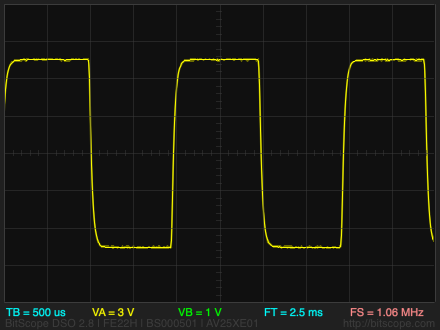For the square wave we take the top byte of the accumulator, and shift it right 7 bits. Because it's a signed integer this gives 0 if the top bit was zero and 0xFF if the top bit was one.

```void Square () {
Acc = Acc + Jump;
int8_t temp = Acc>>8;
OCR1A = temp>>7;
}```

#### Rectangle

The rectangle wave has a duty cycle of 25%: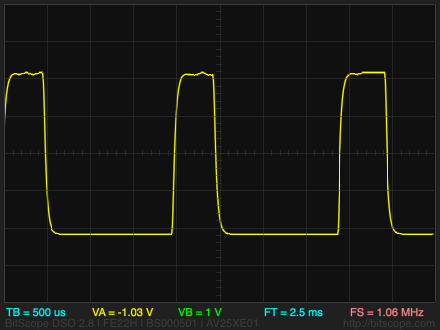A square wave with duty cycle D has a missing harmonic n whenever n*D is an integer, so this wave has the 4th, 8th, 12th harmonics etc missing.

For the rectangle wave we take the top byte of the accumulator, AND together the top two bits, then shift it right 7 bits. This gives 0xFF if the top two bits were one and 0 otherwise.

```void Rectangle () {
Acc = Acc + Jump;
int8_t temp = Acc>>8;
temp = temp & temp<<1;
OCR1A = temp>>7;
}```

#### Pulse

The pulse wave is a rectangle wave with a mark/space ratio of 1:16.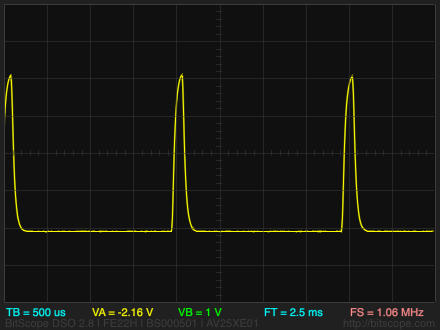For the pulse wave we take the top byte of the accumulator, AND together the top four bits, then shift it right 7 bits. This gives 0xFF if the top four bits were one and 0 otherwise.

```void Pulse () {
Acc = Acc + Jump;
int8_t temp = Acc>>8;
temp = temp & temp<<1 & temp<<2 & temp<<3;
OCR1A = temp>>7;
}```

#### Sawtooth

The sawtooth wave contains all the harmonics. It has an amplitude that counts up from 0 to 255 each cycle, so named because it looks like the teeth of a saw: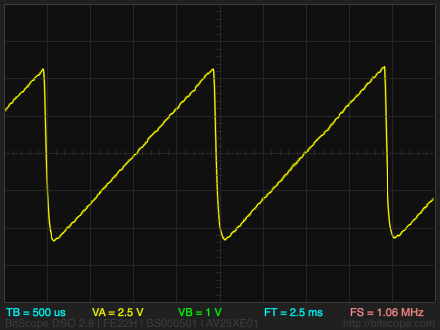For the sawtooth wave we simply copy the top byte of the accumulator to the output:

```void Sawtooth () {
Acc = Acc + Jump;
OCR1A = Acc >> 8;
} ```

#### Triangle

The triangle wave is close to a pure sinewave in shape, but with the addition of odd harmonics at lower levels than the square wave. It counts up from 0 to 255, and then back down to 0 again: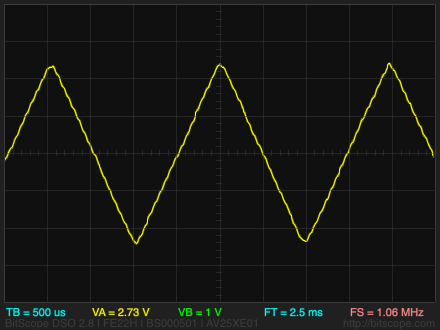To generate the triangle wave we take the top byte of the accumulator, and invert it when the top bit is a one:

```void Triangle () {
Acc = Acc + Jump;
temp = Acc>>8;
OCR1A = temp<<1;
}```

#### Chainsaw

For fun I've included an invented waveform I call chainsaw, which is a sort of cross between a sawtooth wave and a square wave: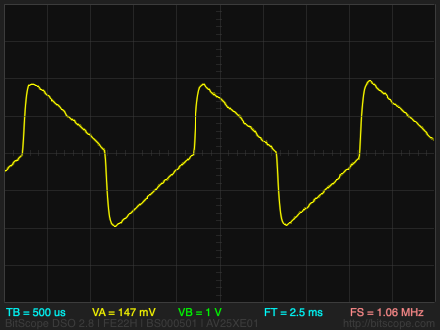Here's how it is generated:

```void Chainsaw () {
Acc = Acc + Jump;
temp = Acc>>8;
top = temp & 0x80;
temp = (temp ^ mask) | top;
OCR1A = temp;
}```

#### Noise

The noise waveform has an even distribution of energy at all frequencies. It isn't affected by the setting of the frequency control.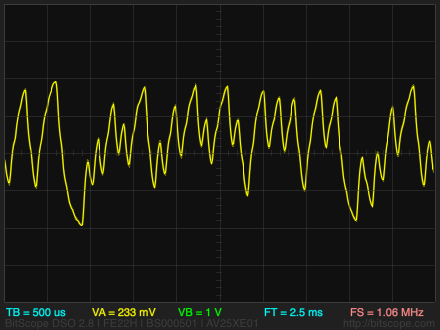The noise waveform uses a pseudo-random number generator to generate random bytes:

```void Noise () {
int8_t temp = Acc & 1;
Acc = Acc >> 1;
if (temp == 0) Acc = Acc ^ 0xB400;
OCR1A = Acc;
}```

### The circuit

Here's the circuit of the Tiny Function Generator: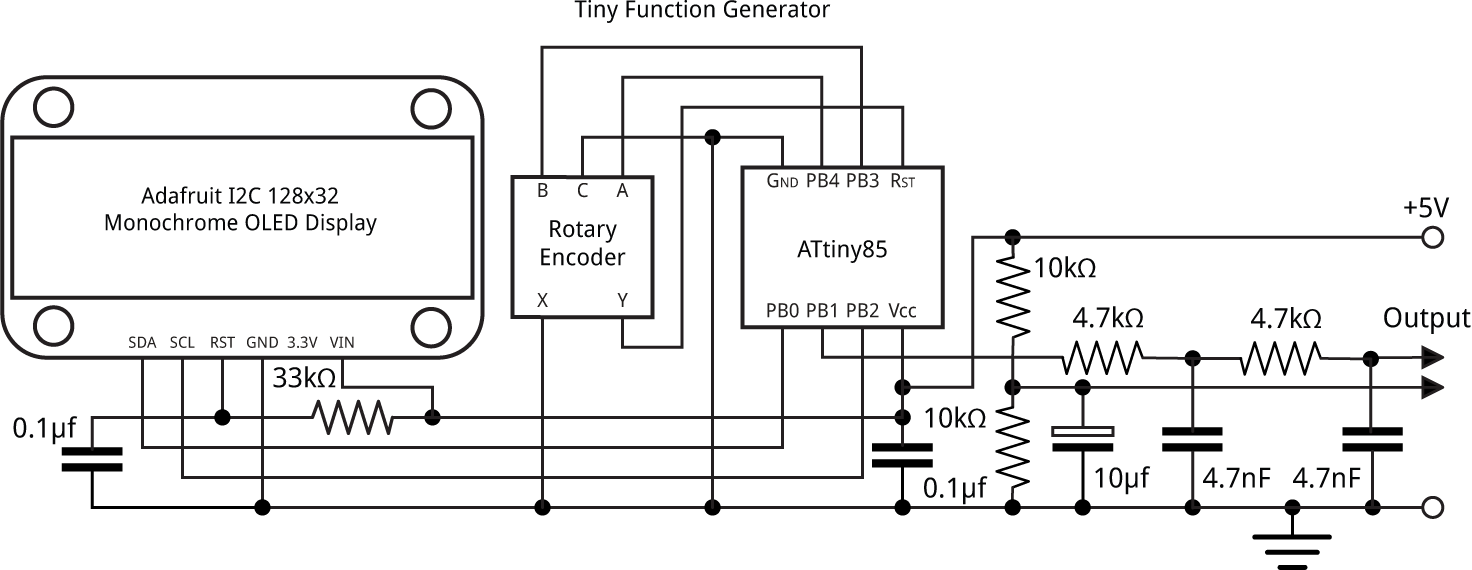Circuit of the Tiny Function Generator based on an ATtiny85.

For the display I chose an I2C 128x32 OLED display available from Adafruit  or Pimoroni in the UK . The 33kΩ resistor and 0.1µF capacitor ensure that the display is reset correctly when power is first applied.

The latest version of Adafruit's 128x32 I2C OLED display includes a reset circuit, so you can omit the 33kΩ resistor and 0.1µF capacitor, but it needs a delay before initialising it, so add a delay(1000) before the InitDisplay() call in setup().

For the rotary encoder I used the Adafruit rotary encoder . This includes a push-switch which can be used to step through the waveforms. The rotary encoder's terminals are slightly too wide to fit into a prototyping board so I soldered a short length of tinned copper wire to each terminal, and inserted these into the breadboard instead.

The 4.7kΩ resistors and 4.7nF capacitors form a two-pole low-pass filter to filter out the PWM carrier frequency. The cutoff of this circuit is 1/2πRC, so these values give a cutoff of 1/(2*3.14*4700*4.7E10-9) which is 7.2kHz. The output is about 1V, adequate to drive an amplifier or a piezo speaker; for a higher output you could use an active filter.

Because the output at PB1 varies between 0V and +5V there's a +2.5V DC offset on the waveforms. The offset is avoided by taking the output relative to a virtual ground created by the two 10kΩ resistors.

### The program

#### Rotary encoder

The rotary encoder is connected to pins 3 and 4, and uses a pin-change interrupt to update the frequency of the function generator. It uses the code from my earlier article Bounce-Free Rotary Encoder, which also gives details of some widely available rotary encoders.

The pin-change interrupt is set up by SetupRotaryEncoder():

```void SetupRotaryEncoder () {
pinMode(EncoderA, INPUT_PULLUP);
pinMode(EncoderB, INPUT_PULLUP);
PCMSK = 1<<EncoderA;        // Configure pin change interrupt on A
GIMSK = 1<<PCIE;            // Enable interrupt
GIFR = 1<<PCIF;             // Clear interrupt flag
}```

Turning the rotary encoder calls ChangeValue(), with a boolean argument to specify the direction. This increments the frequency and updates the OLED display:

```void ChangeValue (bool Up) {
int step = 1;
if (Freq >= 1000) step = 100;
else if (Freq >=100) step = 10;
Freq = max(min((Freq + (Up ? step : -step)), MaxFreq), MinFreq);
PlotFreq(Freq, 1, 7);
Jump = Freq*4;
}```

The Tiny Function Generator can potentially change the frequency from 0.25Hz in steps of 0.25Hz over the whole frequency range, but for convenience I chose to make the frequency change in 1Hz steps between 1Hz and 99Hz, in 10Hz steps between 100Hz and 999Hz, and in 100Hz steps from 1000Hz to 5000Hz, but you could change this.

The rotary encoder switch is used to step between waveforms. Because there aren't any free pins on the ATtiny85 I used this switch to reset the ATtiny85. A variable Wave is used to count the number of resets, and hence select the next waveform; this is defined in the program as .noinit so it will not get set to zero by the compiler on reset. The current frequency, Freq, is also defined as .noinit so it doesn't get initialised on reset.

#### I2C OLED display

The frequency, and an icon representing the current waveform, is displayed on the 128x32 pixel OLED display. This is available in SPI and I2C versions; I chose the I2C version because it only needs 2 I/O lines to drive it, and there are only two lines available on the ATtiny85.

According to the datasheet, the SSD1306 controller used by the display supports sending a mixture of commands and/or data, so in theory you can write the entire display in a single 512-byte I2C transmission. At first I struggled to get this to work. It turns out the Arduino Wire library works by buffering the data you send when you call Wire.write(), and only actually transmits it when you call Wire.endTransmission(). Furthermore, the buffers are only 32 bytes long, so the maximum length of transmission is 32 bytes. One workaround is to write the data and commands as a series of separate one-byte messages, which is what Adafruit do in their SSD1306 library, but this is very inefficient.

My solution was to break up the transmissions into a maximum of 32 bytes at a time. For example, the PlotChar() routine sends 24 bytes for a double-size character, so that can be done in a single transmission.

The frequency and icon are displayed in double-sized characters, to make the display more readable; the technique is described in my earlier article Big Text for Little Display. The characters are defined by the array CharMap[][]. The waveform icons are each created from two characters. I've only included the definitions for the digits, characters "Hz", and the waveform icons in this program, but you can add a full character set if you want to display other characters; get them from my Tiny Terminal.

#### Waveform selection

The most obvious way of allowing you to select a waveform is to use a case statement or a series of if statements to select the section of code for the appropriate waveform depending on the value of a global variable; for example, this would look something like:

```ISR(TIMER0_COMPA_vect) {
if (Wave == 0) {
// Code for triangle wave
} else if (Wave == 1) {
// Code for sawtooth wave
} else if (Wave == 2) {
// Code for square wave
} else {
// Code for noise
}
}
```

However, the Interrupt Service Routine is called 16,000 times a second, so every cycle of execution time is critical, and this approach potentially adds four comparisons to every call. A more elegant solution is to define each of the waveforms as a named subroutine, and then use a lookup table to call the appropriate routine. So, for example, we define the sawtooth as:

```void Sawtooth () {
Acc = Acc + Jump;
OCR1A = Acc >> 8;
}```

We define a type wavefun_t which is a function of no arguments and no return value:

`typedef void (*wavefun_t)();`

and then define an array of the waveform routine addresses with:

```const int nWaves = 4;
wavefun_t Waves[nWaves] = {Triangle, Sawtooth, Square, Noise};```

To change the waveform we execute:

```Wave = (Wave+1) % nWaves;
Wavefun = Waves[Wave];```

The Interrupt Service Routine then becomes:

```ISR(TIMER0_COMPA_vect) {
Wavefun();
}```

### Compiling the program

I compiled the program using Spence Konde's ATTiny Core . Choose the ATtiny25/45/85 option under the ATtinyCore heading on the Board menu. Then choose Timer 1 Clock: CPUB.O.D. DisabledATtiny858 MHz (internal) from the subsequent menus. Choose Burn Bootloader to set the fuses appropriately. Then upload the program using ISP (in-system programming); I used Sparkfun's Tiny AVR Programmer Board; see ATtiny-Based Beginner's Kit.

Here's the whole Tiny Function Generator program: Tiny Function Generator Program.

### Further suggestions

#### Calibrating the frequency

The accuracy of the Tiny Function Generator depends on the accuracy of the ATtiny85's internal 8MHz clock. To get the frequency as accurate as possible you can calibrate the internal clock using the OSCCAL register. The easiest way would be to check the frequency with a frequency meter. If you don't have a frequency meter connect a piezo speaker to the output, select the square wave and a frequency of 1Hz, and count the clicks with a stopwatch! Insert a statement:

`OSCCAL = 128;`

at the start of setup(), and recompile the program with different OSCCAL values, changing it in large jumps at first and then smaller steps as you get closer to the correct frequency.

#### Using the ATtiny861

This application is mainly limited by the number of I/O lines available on the ATtiny85. To extend it you could base it on the ATtiny861 instead, which features the same high-speed PLL clock as the ATtiny85 but provides a more generous 15 I/O lines. This would allow you to provide an external crystal, for more accurate frequency control, and switches to allow more convenient selection of waveform and frequency range.

7th March 2018: I've added a sine wave to the available waveforms: see Tiny Function Generator Sine Wave.

10th March 2018: I've updated the circuit and description to lower the cutoff of the low-pass filter to 7.2kHz, so it's just below half the 16.4kHz sampling rate, the Nyquist frequency. Thanks to John Tuffen for pointing out this error.

23rd September 2019: Added an explanation of how the reset pin is used to step between waveforms.